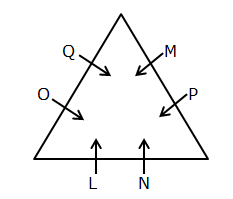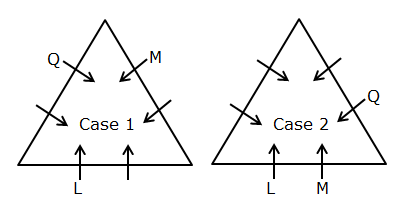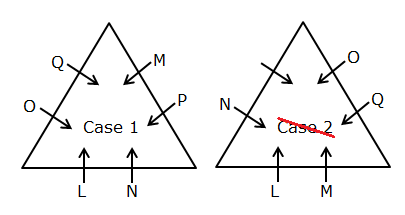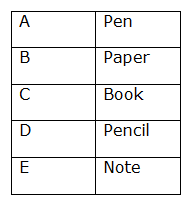# SBI Clerk Prelims Reasoning Ability Questions 2021 (Day-26)

Dear Aspirants, Our IBPS Guide team is providing new series of Reasoning Questions for SBI Clerk Prelims 2021 so the aspirants can practice it on a daily basis. These questions are framed by our skilled experts after understanding your needs thoroughly. Aspirants can practice these new series questions daily to familiarize with the exact exam pattern and make your preparation effective.

Start Quiz

Triangular arrangement

Direction (1-5): Answer the questions based on the information given below.

Six persons viz. L, M, N, O, P and Q are standing on a triangular line such that all of them are facing towards the center, but not necessarily in the same order. Two persons stand on each side of the line.

L stands two persons away from Q. M stands to the immediate left of Q but not on the same side. N stands second to the left of M and second to the right of O. P doesn’t stand adjacent to O.

1) Who among the following person stand to the immediate right of N?

A.M

B.P

C.L

D.O

E.None of these

2) What is the position of O with respect to L?

A.Third to the right

B.Second to the right

C.Second to the left

D.Immediate left

E.Immediate right

3) How many persons stand between Q and P when counted from the right of P?

A.1

B.2

C.3

D.None

E.Cannot be determined

4) Who among the following person stands fourth to the left of O?

A.N

B.L

C.M

D.Q

E.P

5) Fill the following series.

LP ML NO __

A.NQ

B.LP

C.LO

D.PL

E.None of these

Alphabet series

Direction (6-9): Study the following information carefully and answer the below questions

S D T R Y U K L I N G Y D A W Q E C X I Y R G L

6) Which of the following letter will be seventh to the left of the eighth letter from the right end?

A.G

B.N

C.Y

D.I

E.L

7) If all the vowels are arranged after the letter which is third from the right end (following the same order from left to right)then which of the following will be the sixth letter from the right end?

A.E

B.A

C.I

D.U

E.R

8) Find the odd one out?

A.SRY

B.LGY

C.DQE

D.YGL

E.KNG

9) How many consonants are there in the above arrangement each of which is preceding vowels?

A.2

B.3

C.4

D.5

E.More than five

Puzzle

10) Five persons A to E are bought five different items-Book, Pencil, Note, Pen, and Paper in the stationary shop. B bought Paper. C is neither bought Pencil nor Pen. E bought either Paper or note. D does not buyPen. Then which of the following item is bought by A?

A.Book

B.Note

C.Paper

D.Pen

E.Pencil

Directions (1-5):1) L stands two persons away from Q. M stands to the immediate left of Q but not on the same side.2) N stands second to the left of M and second to the right of O. P doesn’t stand adjacent to O.S D T R Y U K L I N G Y D A W Q E C X I Y R G L

S D T R Y K L N G Y D W Q C X Y R U I A EI G L

S D T R Y U K L I N G Y D A W Q E C X I Y R G L

All elements are arranged in S (+3) R (+1) Y form except YGL